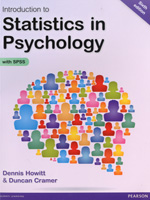Introduction to Statistics in Psychology, 6th EditionBy: Dennis Howitt & Duncan Cramer March 2014 Pearson Education Distributed by Trans-Atlantic Publications Inc. ISBN: 9781292000749 712 Pages, Illustrated \$115.00 Paper original Description: Introduction to Statistics in Psychology is a comprehensive, modern guide to understanding and using statistics in psychological research. This edition has been significantly revised to incorporate the essential SPSS steps you need for carrying out statistical analysis. Contents: 1. Why statistics? Part 1: Descriptive statistics 2. Some basics: Variability and measurement 3. Describing variables: Tables and diagrams 4. Describing variables numerically: Averages, variation and spread 5. Shapes of Distributions of Scores 6. Standard deviation and z-scores: The standard unit of measurement in statistics 7. Relationships between two or more variables: Diagrams and tables 8. Correlation coefficients: Pearson correlation and Spearman’s rho 9. Regression: Prediction with precision Part 2: Significance testing 10. Samples and populations: Generalising and inferring 11. Statistical significance for the correlation coefficient: A practical introduction to statistical inference 12. Standard error: The standard deviation of the means of samples 13. The t-test: Comparing two samples of correlated/related scores 14. The t-test: Comparing two samples of unrelated/uncorrelated scores 15. Chi-square: Differences between samples of frequency data 16. Probability 17. Reporting significance levels succinctly 18. One-tailed versus two-tailed significance testing 19. Ranking tests: Nonparametric statistics Part 3: Introduction to analysis of variance 20. The variance ratio test: The F-ratio to compare two variances 21. Analysis of variance (ANOVA): Introduction to the one-way unrelated or uncorrelated ANOVA 22. Analysis of variance for correlated scores or repeated measures 23. Two-way analysis of variance for unrelated/uncorrelated scores: Two studies for the price of one? 24. Multiple comparisons in ANOVA: Just where do the differences lie? 25. Mixed-design ANOVA: Related and unrelated variables together 26. Analysis of covariance (ANCOVA): Controlling for additional variables 27. Multivariate Analysis of Variance (MANOVA) 28. Discriminant (Function) analysis especially in MANOVA 29. Statistics and the analysis of experiments Part 4: More advanced correlational statistics 30. Partial correlation: Spurious correlation, third or confounding variables, suppressor variables 31. Factor analysis: Simplifying complex data 32. Multiple regression and multiple correlation 33. Path analysis 34. The analysis of a questionnaire/survey project Part 5: Assorted advanced techniques 35. The size of effects in statistical analysis: Do my findings matter? 36. Meta-analysis: Combining and exploring statistical findings from previous research 37. Reliability in scales and measurement: Consistency and agreement 38. Confidence intervals 39. The influence of moderator variables on relationships between two variables 40. Statistical power analysis: getting the sample size right Part 6: Advanced qualitative or nominal techniques 41. Log-Linear Methods: The analysis of complex contingency tables 42. Multinomial logistic regression: Distinguishing between several different categories or groups 43. Binomial Logistic Regression Appendices Appendix A: Testing for excessively skewed distributions Appendix B1: Large sample formulae for the nonparametric tests Appendix B2: Nonparametric tests for three or more groups Appendix C: Extended table of significance for the Pearson correlation coefficient Appendix D: Table of significance for the Spearman correlation coefficient Appendix E: Extended table of significance for the t-test Appendix F: Table of significance for Chi-square Appendix G: Extended table of significance for the sign test Appendix H: Table of significance for the Wilcoxon Matched Pairs Test Appendix I: Table of significance for the Mann-Whitney U-test Appendix J: Table of significance values for the F-distribution Appendix K: Table of significant values oft when making multiple t-tests Glossary References Index